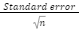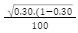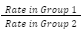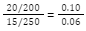# Mastering Statistical Tools: Confidence Intervals, Incidence Rate Ratio, and p-Values for Comparing Two Populations

October 09, 2023Dr. Sarah Anderson
New Zealand
Statistics
Dr. Sarah Anderson holds a Ph.D. in Statistics from the University of Auckland, New Zealand. Her doctoral research focused on advanced statistical modeling techniques for epidemiological studies, solidifying her expertise in the field.

Statistics is an invaluable resource, offering essential assistance with Statistics Assignments using Statistical Tools. Proficiency in statistical concepts and methods is fundamental for both assignment completion and meaningful research endeavors. Within this extensive guide, we will explore three vital statistical instruments frequently encountered in assignments: Confidence Intervals, Incidence Rate Ratio (IRR), and p-Values. Upon completing this article, you will possess a firm understanding of these concepts, empowering you to confidently address assignments that demand their utilization.

## Confidence Intervals

Confidence intervals (CIs) are a fundamental statistical concept used to estimate the range within which a population parameter, such as a mean or proportion, is likely to fall. They provide a measure of uncertainty associated with sample statistics. Confidence intervals are typically expressed as a range with an associated level of confidence, often 95% or 99%.## Constructing Confidence Intervals

To construct a confidence interval, you need three components:

vbnet

1. Point Estimate: This is your best guess at the population parameter based on your sample data. For example, if you are estimating the mean height of a population, your point estimate might be the sample mean height.
2. Margin of Error: This quantifies the uncertainty around your point estimate. It depends on the desired level of confidence and the standard error of your estimate. The formula for the margin of error is:
3. Margin of Error=Z.Where:

Z is the critical value from the standard normal distribution (e.g., 1.96 for 95% confidence).

Standard Error is a measure of the variability in your sample.

n is the sample size.

4. Confidence Level: This represents how confident you are that the true parameter falls within your interval. Common confidence levels are 95% and 99%.

## Interpreting Confidence Intervals

Confidence intervals are like windows into the world of statistics, offering us a glimpse of the uncertainty that surrounds our data-driven estimates. They are not just arbitrary ranges; they are powerful tools that help us navigate the complex landscape of population parameters.

When you see a confidence interval, remember that it doesn't provide a definitive answer but rather a range of plausible values for a parameter, such as a population mean or proportion. The width of the interval represents the level of uncertainty: wider intervals suggest greater uncertainty, while narrower intervals indicate more precise estimates.

Imagine you are estimating the average height of a certain species of trees in a forest. Your sample data gives you a point estimate, which is your best guess at the population mean height based on your sample. However, this estimate is surrounded by a margin of error, which tells you how much your estimate could deviate from the true population mean.

The confidence level associated with the interval, typically 95% or 99%, quantifies our level of confidence that the true population parameter falls within the interval. If we were to draw multiple samples and construct confidence intervals in the same way, our interval would capture the true parameter in approximately 95% (or 99%) of those intervals.

In essence, confidence intervals remind us that the world of statistics is filled with uncertainty. They encourage us to think in terms of probabilities and acknowledge that our data can never provide absolute certainty. Instead, we use these intervals to make informed decisions, weigh evidence, and communicate the reliability of our estimates to others.

So, the next time you encounter a confidence interval in your assignments or research, embrace it as a valuable tool for understanding the inherent uncertainty in statistics and making well-informed conclusions.

## Practical Example - Confidence Intervals in Action

Imagine you are working on a biology assignment, and you want to estimate the proportion of a specific bird species in a forest. You collect data from 100 random locations and find that 30 of them contain the bird species. To estimate the proportion of locations with this species in the entire forest, you can calculate a confidence interval.

Point Estimate (Sample Proportion): 0.30

Confidence Level: 95%

Margin of Error (Z for 95% confidence is approximately 1.96):

Margin of Error=1.96⋅≈0.065

Confidence Interval: 0.30±0.065=(0.235,0.365) at 95% confidence.

This means you are 95% confident that the true proportion of locations with the bird species in the forest falls between 23.5% and 36.5%.

## Applications of Confidence Intervals

Confidence intervals are widely used in various fields, including:

• Healthcare: Estimating the mean blood pressure in a population.
• Marketing: Determining the average customer satisfaction score.
• Finance: Estimating the return on investment (ROI) for a stock.
• Manufacturing: Assessing the defect rate in a production line.

Mastering confidence intervals is essential for making informed decisions and drawing accurate conclusions in these domains.

Incidence Rate Ratio (IRR)

Incidence Rate Ratio (IRR) is a statistic commonly used in epidemiology and medical research to compare the incidence rates of events or outcomes between two groups. It is particularly useful when dealing with count data, such as the number of cases or events in a given time period.

## Calculating IRR

The formula for calculating IRR is as follows:

IRR=Where:

Rate in Group 1 is the incidence rate of the event in the first group.

Rate in Group 2 is the incidence rate of the event in the second group.

## Interpreting IRR

• IRR = 1: The event rates in both groups are equal.
• IRR > 1: The event rate in Group 1 is higher than in Group 2.
• IRR < 1: The event rate in Group 1 is lower than in Group 2.

A key advantage of IRR is its ability to compare event rates between groups while accounting for differences in exposure or follow-up time.

## Practical Example - IRR in Epidemiology

Let's say you're conducting a study on the effectiveness of two different treatments for a certain disease. You have two groups: Group A, treated with Treatment A, and Group B, treated with Treatment B. You want to compare the incidence rates of disease relapse in both groups over a one-year period.

Group A: 20 relapses in 200 person-years of follow-up.

Group B: 15 relapses in 250 person-years of follow-up.

Calculate the IRR to compare the incidence rates:

IRR =≈1.67

The IRR of approximately 1.67 indicates that the incidence rate of disease relapse is 1.67 times higher in Group A (Treatment A) compared to Group B (Treatment B) over the one-year period.

## Applications of IRR

Incidence Rate Ratio is commonly used in epidemiology to compare disease rates between different populations, study groups, or time periods. Researchers and public health professionals use IRR to:

• Assess the impact of interventions on disease incidence.
• Examine the risk factors associated with disease development.
• Compare disease rates across regions or demographic groups.

Mastering IRR is crucial for making informed decisions in public health and epidemiological research.

p-Values

p-Values are a statistical tool used to determine the strength of evidence against a null hypothesis. The null hypothesis typically represents a statement of no effect or no difference between groups. By calculating a p-value, you can assess whether the observed results are statistically significant.

## How p-Values Work

In the realm of statistics, p-values serve as a critical tool for gauging the strength of evidence against a null hypothesis. However, to fully grasp their significance, one must delve into the inner workings of this statistical metric.

At its core, a p-value quantifies the probability of obtaining results as extreme as, or even more extreme than, those observed—assuming that the null hypothesis is valid. In simpler terms, it answers a fundamental question: "How likely is it to encounter these results if there's no real effect or difference between groups in the population?"

Imagine you're conducting a study to evaluate the efficacy of a new medication in reducing pain levels among patients. You set up a null hypothesis (H0) that asserts there's no meaningful difference in pain reduction between the medication and a placebo. Conversely, your alternative hypothesis (Ha) posits that the medication indeed has a significant impact.

You collect data, run your analysis, and calculate a p-value of 0.03. Now, what does this p-value signify? In this context, a p-value of 0.03 means that if the null hypothesis were entirely accurate—meaning there's truly no difference between the medication and placebo—you would only observe results as extreme as those you collected about 3% of the time purely by random chance.

The significance of the p-value becomes evident when you introduce a significance level, typically set at 0.05. If your p-value is less than this threshold (p < 0.05), it suggests that the observed results are quite unlikely to occur purely due to random variation. Consequently, you may decide to reject the null hypothesis, indicating that there's substantial evidence supporting the alternative hypothesis. In essence, you're saying that the medication likely has a genuine effect.

## Interpreting p-Values

• p < 0.05: Typically considered statistically significant. It suggests that the observed results are unlikely to occur by random chance, and you may reject the null hypothesis.
• p ≥ 0.05: Not statistically significant. It suggests that the observed results could reasonably occur by random chance, and you fail to reject the null hypothesis.

It's crucial to remember that a p-value does not prove the null hypothesis is true or false; it merely assesses the strength of evidence against it.

## Practical Example - Hypothesis Testing with p-Values

Suppose you are conducting a psychology experiment to test whether a new therapy reduces anxiety levels in participants. You have two groups: Group X, which receives the therapy, and Group Y, which does not. You collect anxiety scores from both groups and perform a t-test to compare the means.

• Null Hypothesis (H0): The therapy has no effect; the mean anxiety scores in both groups are equal.
• Alternative Hypothesis (Ha): The therapy reduces anxiety; the mean anxiety score in Group X is lower than in Group Y.

You calculate a p-value of 0.02. Since p < 0.05 (typically chosen significance level), you reject the null hypothesis.

## Applications of p-Values

p-Values are a fundamental tool in hypothesis testing and statistical inference. They are widely used in various fields, including:

• Clinical Trials: Assessing the efficacy of new drugs.
• Social Sciences: Analyzing survey data to identify significant associations.
• Quality Control: Ensuring product quality by comparing sample data to specified standards.
• Environmental Studies: Investigating the impact of pollution on ecosystems.

Mastering p-values is essential for drawing meaningful conclusions from data and making informed decisions in research and industry.

Comparing Two Populations Using Statistics

Now that we understand Confidence Intervals, Incidence Rate Ratio, and p-Values individually, let's explore how to use them to compare two populations effectively.

## Hypothesis Testing

To compare two populations, you can set up a hypothesis test using p-values. Here's a general framework:

1. Null Hypothesis (H0): The two populations are the same (e.g., the means or proportions are equal).
2. Alternative Hypothesis (Ha): The two populations are different (e.g., the means or proportions are not equal).
3. Choose a significance level (usually 0.05).
4. Collect data from both populations and perform the appropriate statistical test (e.g., t-test, chi-square test, or regression analysis).
5. Calculate the p-value.
6. Compare the p-value to the chosen significance level.
7. If p < 0.05, you reject the null hypothesis, indicating a significant difference between the populations.

## Confidence Intervals for Comparing Means

To compare means between two populations, calculate confidence intervals for each population's mean. If the intervals overlap, it suggests no significant difference. If they don't overlap, it suggests a potential difference.

### Incidence Rate Ratio for Comparing Event Rates

difference in event rates. If IRR > 1, Group 1 has a higher event rate, and if IRR < 1, Group 2 has a higher event rate.

## Conclusion

In this extensive guide, we've explored three vital statistical concepts: Confidence Intervals, Incidence Rate Ratio, and p-Values. Understanding these tools and how to apply them is crucial for students tackling assignments that involve comparing two populations or estimating population parameters.

Mastering these statistical techniques will not only help you excel in your assignments but also provide you with valuable skills that are applicable in various research and data analysis endeavors. So, embrace the power of statistics, and let these tools guide you towards evidence-based conclusions and meaningful insights. Whether you're exploring the proportions of bird species in a forest, comparing disease incidence rates, or testing the effectiveness of a therapy, these statistical tools will be your companions in the world of data analysis and decision-making.